# US8488726B2 - Receivers based on closed-form parametric estimates of the probability density function for the received signal - Google Patents

## Info

Publication number
US8488726B2
US8488726B2 US11/832,585 US83258507A US8488726B2 US 8488726 B2 US8488726 B2 US 8488726B2 US 83258507 A US83258507 A US 83258507A US 8488726 B2 US8488726 B2 US 8488726B2
Authority
US
United States
Prior art keywords
channel
noise
signal
Prior art date
Legal status (The legal status is an assumption and is not a legal conclusion. Google has not performed a legal analysis and makes no representation as to the accuracy of the status listed.)
Active, expires
Application number
US11/832,585
Other versions
US20120275780A1 (en
Inventor
Mario R. Hueda
Diego E. Crivelli
Hugo S. Carrer
Oscar E. Agazzi
Current Assignee (The listed assignees may be inaccurate. Google has not performed a legal analysis and makes no representation or warranty as to the accuracy of the list.)
Inphi Corp
Original Assignee
Clariphy Communications Inc
Priority date (The priority date is an assumption and is not a legal conclusion. Google has not performed a legal analysis and makes no representation as to the accuracy of the date listed.)
Filing date
Publication date
Priority to US82113706P priority Critical
Application filed by Clariphy Communications Inc filed Critical Clariphy Communications Inc
Priority to US11/832,585 priority patent/US8488726B2/en
Assigned to CLARIPHY COMMUNICATIONS, INC. reassignment CLARIPHY COMMUNICATIONS, INC. ASSIGNMENT OF ASSIGNORS INTEREST (SEE DOCUMENT FOR DETAILS). Assignors: CARRER, HUGO S., AGAZZI, OSCAR E., CRIVELLI, DIEGO E., HEUDA, MARIO R.
Publication of US20120275780A1 publication Critical patent/US20120275780A1/en
Publication of US8488726B2 publication Critical patent/US8488726B2/en
Application granted granted Critical
Assigned to INPHI CORPORATION reassignment INPHI CORPORATION ASSIGNMENT OF ASSIGNORS INTEREST (SEE DOCUMENT FOR DETAILS). Assignors: CLARIPHY COMMUNICATIONS, INC.
Application status is Active legal-status Critical

• 230000001419 dependent Effects 0 abstract claims description 8
• 230000003287 optical Effects 0 abstract description 56
• 230000014509 gene expression Effects 0 abstract description 20
• 230000001131 transforming Effects 0 claims description 16
• 230000001186 cumulative Effects 0 claims description 15
• 239000006185 dispersions Substances 0 claims description 15
• 238000000034 methods Methods 0 abstract description 13
• 230000000875 corresponding Effects 0 abstract description 8
• 230000000051 modifying Effects 0 claims description 7
• 230000000694 effects Effects 0 claims description 5
• 239000003365 glass fiber Substances 0 claims description 5
• 238000005315 distribution function Methods 0 claims 12
• 238000005516 engineering processes Methods 0 description 7
• 238000004458 analytical methods Methods 0 description 5
• 230000015654 memory Effects 0 description 5
• 239000007787 solids Substances 0 description 5
• 238000004422 calculation algorithm Methods 0 description 4
• 230000001808 coupling Effects 0 description 4
• 238000010168 coupling process Methods 0 description 4
• 238000005859 coupling reaction Methods 0 description 4
• 239000000835 fiber Substances 0 description 4
• 239000000203 mixtures Substances 0 description 4
• 230000001702 transmitter Effects 0 description 4
• 238000007476 Maximum Likelihood Methods 0 description 3
• 238000005094 computer simulation Methods 0 description 3
• 230000000996 additive Effects 0 description 2
• 239000000654 additives Substances 0 description 2
• 230000006399 behavior Effects 0 description 2
• 238000009826 distribution Methods 0 description 2
• 230000001965 increased Effects 0 description 2
• 230000002829 reduced Effects 0 description 2
• 230000003044 adaptive Effects 0 description 1
• 239000002004 ayurvedic oil Substances 0 description 1
• 238000004364 calculation methods Methods 0 description 1
• 230000015556 catabolic process Effects 0 description 1
• 230000001721 combination Effects 0 description 1
• 230000000295 complement Effects 0 description 1
• 230000004059 degradation Effects 0 description 1
• 238000006731 degradation Methods 0 description 1
• 230000001976 improved Effects 0 description 1
• 239000011133 lead Substances 0 description 1
• 230000000670 limiting Effects 0 description 1
• 239000002609 media Substances 0 description 1
• 150000004706 metal oxides Chemical class 0 description 1
• 229910044991 metal oxides Inorganic materials 0 description 1
• 238000002156 mixing Methods 0 description 1
• 230000004048 modification Effects 0 description 1
• 238000006011 modification Methods 0 description 1
• 230000000644 propagated Effects 0 description 1
• 230000001603 reducing Effects 0 description 1
• 238000006722 reduction reaction Methods 0 description 1
• 230000004044 response Effects 0 description 1
• 238000005070 sampling Methods 0 description 1
• 239000004065 semiconductor Substances 0 description 1
• 230000002269 spontaneous Effects 0 description 1
• 238000002945 steepest descent method Methods 0 description 1
• 230000036962 time dependent Effects 0 description 1
• 230000035899 viability Effects 0 description 1

## Images

•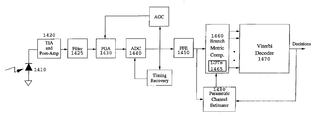•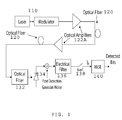•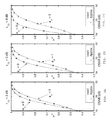•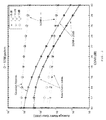•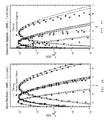•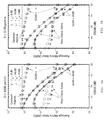•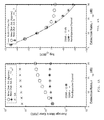•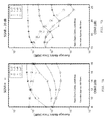•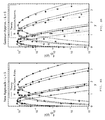•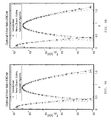•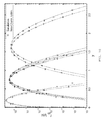•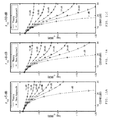•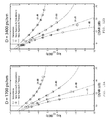•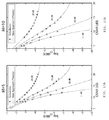•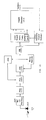•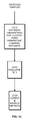## Classifications

• HELECTRICITY
• H04ELECTRIC COMMUNICATION TECHNIQUE
• H04LTRANSMISSION OF DIGITAL INFORMATION, e.g. TELEGRAPHIC COMMUNICATION
• H04L25/00Baseband systems
• H04L25/02Details ; Arrangements for supplying electrical power along data transmission lines
• H04L25/03Shaping networks in transmitter or receiver, e.g. adaptive shaping networks ; Receiver end arrangements for processing baseband signals
• H04L25/03006Arrangements for removing intersymbol interference
• H04L25/03178Arrangements involving sequence estimation techniques
• H04L25/03248Arrangements for operating in conjunction with other apparatus
• H04L25/03292Arrangements for operating in conjunction with other apparatus with channel estimation circuitry
• HELECTRICITY
• H04ELECTRIC COMMUNICATION TECHNIQUE
• H04LTRANSMISSION OF DIGITAL INFORMATION, e.g. TELEGRAPHIC COMMUNICATION
• H04L25/00Baseband systems
• H04L25/02Details ; Arrangements for supplying electrical power along data transmission lines
• H04L25/0202Channel estimation

## Abstract

A closed-form parametric approach to channel-estimation is provided. In one aspect, a specific parametric expression is presented for the received signal pdf that accurately models the behavior of the received signal in IM/DD optical channels. The corresponding parametric channel-estimation approach simplifies the design of MLSE receivers. The general technique lends itself well to the estimation of the signal pdf in situations where there are multiple sources of noise with different distributions, such as ASE noise, together with Gaussian and quantization noise, and signal-dependent noise, for example.

## Description

CROSS-REFERENCE TO RELATED APPLICATION(S)

This application claims priority under 35 U.S.C. §119(e) to U.S. Provisional Patent Application Ser. No. 60/821,137, “Parametric estimation of IM/DD optical channels using new closed-form approximations of the signal pdf,” filed Aug. 2, 2006. The subject matter of the foregoing is incorporated herein by reference in its entirety.

BACKGROUND OF THE INVENTION

1. Field of the Invention

This invention relates generally to receivers and, more specifically, to receivers that use closed-form parametric estimates of the channel and/or received signal probability density function.

2. Description of the Related Art

In many situations, receiver performance depends on knowledge about the received signal and/or the channel over which the signal has propagated. For example, in the case of intensity-modulation/direct-detection (IM/DD) optical-transmission systems at speeds of 10 Gb/s and higher, chromatic dispersion and polarization-mode dispersion have become major factors that limit the reach of these systems. Electronic dispersion compensation (EDC) is an increasingly popular approach to mitigate these impairments and a cost-effective alternative to purely optical-dispersion-compensation techniques.

Among EDC techniques, maximum-likelihood sequence estimation (MLSE) is a promising approach. MLSE chooses the sequence that minimizes the negative logarithm of the likelihood function (i.e., the metric). However, MLSE receivers require knowledge of the statistics of the noisy received signal. Noise in IM/DD optical channels is strongly non-Gaussian and signal dependent. Except in the simplest situations, the pdf of the signal corrupted by noise does not have a closed-form expression. This can lead to difficulties in the implementation of the MLSE receiver.

If the signal pdf is not known a priori by the receiver, it must be estimated based on the received signal. This is a process known as channel-estimation. In an EDC receiver implemented as a monolithic integrated circuit, channel-estimation algorithms typically must be implemented by dedicated hardware. The amount of computational resources that can be devoted to channel-estimation is usually limited by constraints on the chip area and power dissipation. Therefore, finding computationally efficient channel-estimation methods is of paramount importance.

Channel-estimation methods can be parametric or nonparametric. Parametric methods assume that the functional form for the pdf of the signal is known but its parameters are not, whereas nonparametric methods do not assume any knowledge of the pdf. The main difficulty with nonparametric methods is that a large number of samples are needed to obtain accurate estimates. This is particularly problematic in the tail regions of the signal pdf, where it may take an inordinate amount of time to obtain enough samples. For this reason, parametric methods are preferable. However, parameter estimation may be difficult if the functional form assumed for the pdf is cumbersome or does not have a closed-form expression, particularly when the estimation must be done by hardware operating in real time, as in the case of an adaptive EDC receiver.

Thus, there is a need for improved, computationally efficient approaches to channel estimation and receivers that depend on channel-estimation.

SUMMARY OF THE INVENTION

The present invention overcomes the limitations of the prior art by providing a closed-form parametric approach to channel-estimation. In one aspect, a specific parametric expression is presented for the received signal pdf that accurately models the behavior of the received signal in IM/DD optical channels. The corresponding parametric channel-estimation approach simplifies the design of MLSE receivers. The general technique lends itself well to the estimation of the signal pdf in situations where there are multiple sources of noise with different distributions, such as ASE noise, together with Gaussian and quantization noise, and signal-dependent noise, for example.

Another aspect of the invention is the computation of bit-error rates (BER), for example for MLSE receivers operating on IM/DD channels. As a specific example, a closed-form analytical expression for the bit-error probability of MLSE-based receivers in dispersive optical channels in the presence of ASE noise and post detection Gaussian noise is presented. Analytical expressions of the BER are useful not only to predict system performance, but also to facilitate the design of channel codes. Numerical simulations demonstrate the accuracy of the closed-form parametric expressions.

One aspect of the invention includes a receiver based on closed-form parametric channel estimation. In one specific embodiment, the receiver includes a parametric channel-estimator, a branch-metric computation unit and a decoder (e.g., a Viterbi decoder). The parametric channel-estimator provides a channel-estimate based on a closed-form parametric model of the channel. The parameters for the model are estimated based on the received signal. The branch-metric computation unit determines branch metrics for each of the possible received bit sequences based in part on the channel-estimate from the parametric channel-estimator, and the decoder determines the received bit sequence based in part on the branch metrics from the branch-metric computation unit. One advantage of closed form parametric estimates is that they typically require fewer computational resources to implement. The parametric approach can also be used in other embodiments for other channels (e.g., noise sources) and/or receiver architectures (e.g., different types of decoders).

Other aspects of the invention include methods and systems corresponding to the devices described above.

BRIEF DESCRIPTION OF THE DRAWINGS

The invention has other advantages and features which will be more readily apparent from the following detailed description of the invention and the appended claims, when taken in conjunction with the accompanying drawings, in which:

FIG. 1 is a block diagram of an example system suitable for use with the present invention.

FIGS. 2A-2C are graphs of parameter vs, as a function of OSNR for different values of r10.

FIG. 3 is a graph of AME as a function of OSNR, for various channel estimation methods, values of SGNR and values of r10.

FIGS. 4A-4B are graphs of the signal pdf, for various worst case scenarios.

FIGS. 5A-5B are graphs of AME as a function of OSNR, for various channel estimation methods, values of SGNR and values of r10.

FIG. 6A is a graph of AME as a function of the extinction-ratio r10 for various channel estimation methods.

FIG. 6B is a graph of BER as a function of r10 for various channel estimation methods.

FIGS. 7A-7B are graphs of AME as a function of OSNR, for various channel estimation methods, values of SGNR and values of L.

FIGS. 8A-8B are graphs of signal pdf's for the parametric channel estimate and Gaussian approximation, respectively.

FIGS. 9A-9B are graphs of signal pdf's for various channel estimation methods, using realistic filters.

FIG. 10 is a further graph of signal pdf's according to the invention.

FIGS. 11A-11C are graphs of log(BER) as a function of OSNR, for various values of SGNR and r10.

FIGS. 12A-12B are graphs of log(BER) as a function of OSNR, for various values of SGNR and D.

FIGS. 13A-13B are graphs of log(BER) as a function of OSNR, for various values of SGNR and M.

FIG. 14 is a block diagram of an MLSE receiver according to the present invention.

FIG. 15 is a flow diagram for updating the LUTs shown in FIG. 14.

The figures depict embodiments of the present invention for purposes of illustration only. One skilled in the art will readily recognize from the following discussion that alternative embodiments of the structures and methods illustrated herein may be employed without departing from the principles of the invention described herein.

DETAILED DESCRIPTION OF THE PREFERRED EMBODIMENTS

Outline

• 1. MLSE in IM/DD Optical Channels
• 1.A. A Closed-Form Approximation for the Signal PDF
• 2. Example Closed-Form Parametric Expression for the Signal PDF
• 2.A. Approximating Ts(•) in IM/DD Optical Channels
• 2.B. Channel-estimation
• 2.C. On the Gaussian Approximation (GA)
• 3. Accuracy of the Parametric Expression for Signal PDF (22)
• 3.A. Measure of the Goodness of PDF Approximations for Metrics Evaluation
• 3.B. Channel Estimation in the Presence of Ideal Filters
• 3.C. Channel Estimation in the Presence of Ideal Filters and Quantization
• 3.D. Channel Estimation in the Presence of Realistic Filters
• 4. Performance of MLSE in IM/DD Optical Channels
• 4.A. Closed-Form Approximation for the Error-Event Probability in Dispersive IM/DD Channels
• 4.B. Numerical Results
• 5. Example Implementations
• 5.A. Practical Implementation using the Method of Moments
• 6. Further Examples
1. MLSE in IM/DD Optical Channels

Various aspects of the invention will first be introduced in the context of MLSE receivers for IM/DD systems. FIG. 1 shows a simplified model of such a system. The transmitter 110 modulates the intensity of the transmitted signal using a binary alphabet (e.g., ON-OFF-keying (OOK) modulation). Let {an}=(a1, a2, . . . , aN) and N denote, respectively, the bit sequence to be sent on the optical fiber 120 (anε{0,1}) and the total number of transmitted symbols. The optical power ratio between the pulses representing a logical 1 and a logical 0, r10, is called the extinction ratio. Assume that the intensity level for a logical 0 (an=0) is different from zero, which is usual in practical transmitters (e.g., r01=r10 −1≈0.1). The optical fiber introduces chromatic dispersion and polarization mode dispersion, as well as attenuation. Optical amplifiers 122 are deployed periodically along the fiber 120 to compensate the attenuation, also introducing ASE (amplified spontaneous emission) noise in the signal. ASE noise is modeled as additive white Gaussian noise in the optical domain. At the receiver 130 (elements 132-140), the optical signal is filtered by optical filter 132 and then converted to a current with a p-i-n diode 134 or avalanche photodetector 134. The resulting photocurrent is filtered by an electrical filter 136. For simplicity, in the following analysis, we use an ideal low-pass optical filter 132 and an integrate-and dump electrical filter 136. The output of the filter 136 is ideally sampled 138 (i.e., with infinite resolution) at the symbol rate 1/T and applied to the MLSE 140.

The received samples can be written as
y n =s n +r n +z n =x n +z n  (1)
where sn=ƒ(an, . . . , an−δ+1) is the noise-free signal in the electrical domain, which is, in general, a nonlinear function of a group of δ consecutive transmitted bits (note that snεS={ s 0, s 1, . . . , s k} with s 0=ƒ(0, 0 . . . , 0), s 1=ƒ(0, 0 . . . , 1), . . . , s k=ƒ(1, 1 . . . , 1)) where K=2δ−1; rn are samples of the ASE noise in the electrical domain; xn=sn+rn; and zn are samples of the electrical (thermal) noise, which is modeled as a zero-mean Gaussian random process.

Then, the pdf's of x and z can be expressed as

$f x | s ⁡ ( x | s ) = 1 N 0 ⁢ ( x s ) M - 1 2 ⁢ ⅇ - x + s N 0 ⁢ I M - 1 ⁡ ( 2 ⁢ x ⁢ ⁢ s N 0 ) ( 2 ) f z ⁡ ( z ) = 1 2 ⁢ πσ e ⁢ ⅇ - z 2 2 ⁢ σ e 2 ( 3 )$
where σe 2 is the power of z, N0 is related to the variance of the ASE noise in the optical domain, M is the ratio of the optical to electrical bandwidth of the receiver, and Im(•) is the mth modified Bessel function of the first kind For convenience, the time index n is omitted. Note that the ASE noise component in the polarization orthogonal to the signal is neglected in (2) and that the chi-square pdf for the ASE noise is not exact in the presence of practical optical/electrical filters. However, the analysis and assumptions presented in the following are still valid, even when practical filters are used.

Since the noise components r and z are independent random variables, the conditional pdf of y can be obtained from (2) and (3) as follows:
ƒy|s(y|s)=ƒx|s(y|s)

ƒz(y), sεS  (4)
where denotes convolution.

1.A. A Closed-Form Approximation for the Signal PDF

The signal pdf (4) does not have a closed-form analytical expression. However, when r01>0 and N0 is sufficiently small, it can be approximated using the following expression for (2):

$f x | s ⁡ ( x | s ) ≈ 1 2 ⁢ π ⁢ s ~ ⁢ N 0 ⁢ ⅇ - ( x - s ~ ) 2 N 0 ( 5 )$
with x≧0 and
{tilde over (s)}=E s {x}=s+I sp  (6)
where Es{•} denotes conditional expectation given the noise-free signal level s, and Isp=Es{rn}=N0M. When N0<<sn, notice that {tilde over (s)}≈s. Then, using (5) in pdf (4) and applying the method of steepest descent to approximate the convolution integral, the pdf (4) can be expressed as:

$f y | s ⁡ ( y | s ) ≈ G s ⁢ ⅇ - g s ⁡ ( y ) ( 7 ) g s ⁡ ( y ) = ( w - s ) 2 N 0 + ( y - w ) 2 2 ⁢ σ e 2 ( 8 )$
where Gs, is a signal-dependent factor such ∫−∞ Gse−g s (y)dy=1, while ω>0 is the value that minimizes (8).

The maximum-likelihood sequence receiver for signals affected by nonlinear intersymbol interference and additive Gaussian noise consists of a matched-filter bank followed by a Viterbi decoder. It is known that in the case of Gaussian noise, samples of the signal taken at the output of the matched filter at the symbol rate constitute a set of sufficient statistics for the detection. In the case of non-Gaussian and signal-dependent noise, the problem of obtaining a set of sufficient statistics by sampling a filtered version of the input signal at the symbol rate has not been solved. In the following, we assume that the output of the photodetector is filtered and then sampled at the symbol rate, but we do not assume that the input filter is a matched filter bank. We assume that the samples of the signal plus noise are independent, but they are not identically distributed.

The MLSE receiver chooses, among the 2N possible sequences, the one {ân}=(â1, . . . , âN) that minimizes the cumulative metric

$M = ∑ n = 1 N ⁢ ⁢ - ln ⁡ ( f y | s ⁡ ( y n | s ^ n ) ) ( 9 )$
where ŝn=ƒ(ân, . . . , ân−δ+1). The minimization can be efficiently implemented using the Viterbi algorithm. Whereas in Gaussian channels, the branch metrics are simple Euclidean distances; in these optical channels, the branch metrics require the evaluation of different functions for each branch. This is the result of the fact that the noise is signal dependent. In general, the functions representing the branch metrics do not have a closed-form analytical expression.
2. Example Closed-Form Parametric Expression for the Signal PDF

It is well known from the literature that the random variable y can be transformed into a Gaussian random variable u by using a nonlinear transformation Ts(•) as follows:
u=T s(y)=F u|s (−1)(F y|s(y))  (10)
where Fu|s(•) and Fy|s(•) are the cumulative distribution functions of u and y, respectively, when the noise-free signal s is received. From (10) it is possible to show that

$f y | s ⁡ ( y | s ) = 1 2 ⁢ πς s ⁢ ⅇ - 1 2 ⁢ ς s ⁡ [ T s ⁡ ( y ) - u _ s ] 2 ⁢ T s ′ ⁡ ( y ) , ∀ y . ( 11 )$
where T′s(y)=dTs(y)/dy, while ūs and ζs and are the mean and variance of u, respectively.

Suppose that y is concentrated near its means {tilde over (s)} so ƒy|s(y|s) is negligible outside an interval ({tilde over (s)}−ε, {tilde over (s)}+ε) with ε>0, and in this interval, T′s(y)≈T′s({tilde over (s)}). From the Chebyshev inequality, note that this condition can be verified when the noise power is sufficiently low, that is, Pr{|y−{tilde over (s)}|≧ε}≦(M2,s2) with M2,s being the conditional second-order central moment of the received signal y. Thus, we can verify that
E s {y}={tilde over (s)}≈s  (12)
ū s ≈T s({tilde over (s)})≈T s(s)  (13)
ζs ≈[T′ s({tilde over (s)})]2 M 2,s ≈[T′ s(s)]2 M 2,s  (14)
T′ s(y)≈T′ s(s)  (15)
Using (13)-(15), it is simple to show that the generic pdf (11) can be approximated by

$f y | s ⁡ ( y | s ) ≈ 1 2 ⁢ π ⁢ ⁢ M 2 , s ⁢ ⅇ - 1 2 ⁢ ς s ⁡ [ T s ⁡ ( y ) - T s ⁡ ( s ) ] 2 ⁢ s ∈ S . ( 16 )$

Based on (16), we can verify that minimizing the cumulative metric M from (9) is equivalent to minimizing

$M ^ = ∑ n - 1 N ⁢ ⁢ 1 2 ⁢ ς s n ⁡ [ T s ^ n ⁡ ( y n ) - T s ^ n ⁡ ( s ^ n ) ] 2 + 1 2 ⁢ ln ⁡ ( 2 ⁢ π ⁢ ⁢ M 2 , s ^ n ) . ( 17 )$
From (13) and (14) note that, once Ts(•) is known, all parameters required to evaluate (17) can be directly estimated from the input samples.

2.A. Approximating Ts(•) in IM/DD Optical Channels

The exact conditional pdf of the received signal can be written as
ƒy|s(y|s)=G s e −g s (y)  (18)
where Gs is a normalization factor, and gs(•) is a given function. In IM/DD optical channels with combined ASE noise and Gaussian noise, gs(•) can be accurately approximated by (8). From (16) and (18), note that

$g s ⁡ ( y ) ≈ 1 2 ⁢ ς s ⁡ [ T s ⁡ ( y ) - T s ⁡ ( s ) ] 2 . ( 19 )$

In general, obtaining a simple analytical expression for Ts(•) from (10) or (19) is difficult. However in most cases of interest, it is possible to derive a good approximation by analyzing the properties of gs(•) and Ts(•). Assuming that Ts(•) is a differentiable increasing function, it can be approximated by
T s(y)≈H Θs(y), sεS  (20)
where HΘs(y) is a given differentiable increasing function with unknown parameters defined by the set Θs. Additionally, it can be shown that in optical channels with combined ASE noise and post-detection Gaussian noise, Ts(•) is a concave function. Thus, from (20), we conclude that function HΘs(•) should also be concave. Assuming that r01>0 and the signal-to-noise ratio (SNR) is sufficiently high (i.e., Pr{yn<0}≈0)), we have found that the set of parametric concave functions defined by
H Θs(y)=y v s , 0<v s≦1  (21)
with Θs={vs} is adequate to accurately approximate Ts(y) in transmissions over optical channels. Note that a linear function (vs=1) is both concave and convex. Using (12)-(16) and (21), we obtain the following closed form parametric approximation for the pdf of the received signal:

$f y | s ⁡ ( y | s ) = v s ⁢ s ( v s - 1 ) 2 ⁢ π ⁢ ⁢ ς s ⁢ ⅇ - 1 2 ⁢ ς s ⁢ ( y v s - s v s ) 2 , s ∈ S . ( 22 )$
where ζs and vs are defined by (11) and (21).

For combined ASE noise and post-detection Gaussian noise (σe>0, N0>0), note that Pr{y≦0}>0; thus approximation (21) may not be defined if vs<1. As we shall show later, this problem can be overcome by adding an appropriate constant yc to the input signal and neglecting the negative values of y+yc.

2.B. Channel-estimation

Parameters N0, σe 2, M, and set S can be obtained by using the method of moments (e.g., see O. E. Agazzi, et al., “Maximum Likelihood Sequence Estimation in Dispersive Optical Channels,” J. Lightwave Technology, vol. 23 no. 2, pp. 749-763, February 2005, which is incorporated by reference herein). The sets of parameters {vs} and {ζs} with sεS can be calculated as follows. Since u=yv s is approximately a Gaussian random variable with mean ūs, its third-order central moment ηs should be zero for the optimal value of vs, that is
ηs =E{(y v s −ū s)3}=0.  (23)
A simple iterative algorithm can be used to look for the value of vs that minimizes |ηs|. The third-order central moment ηs can be numerically evaluated by using the signal pdf (4).

In the case of IM/DD optical channels with combined ASE noise and Gaussian noise, vs can be estimated directly from the central moments of y as follows:

$v s ≈ 1 - 2 3 ⁢ sM 3 , s M 4 , s - M 2 , s 2 , s ∈ S ( 24 )$
where M3,s and M4,s are the conditional third and fourth-order central moments of the received signal y, respectively. From (24), both Gaussian noise (σe>0, N0=0) and ASE noise (σe=0, N0>0) are special cases of (22):

$Gaussian ⁢ ⁢ noise ⁢ : ⁢ ⁢ ς s = σ e 2 , v s = 1 , y ∈ ℜ ⁢ ⁢ ASE ⁢ ⁢ noise ⁢ : ⁢ ⁢ ς s = N 0 2 , v s = 0.5 , y > 0. ( 25 )$

Once vs is estimated, parameters ζs and ūs can be obtained from (13) and (14) as follows:
ū s ≈s v s   (26)
ζs ≈v s 2 s 2(v s −1) M 2,s.  (27)

2.C. On the Gaussian Approximation

When the SNR is sufficiently high, the term between brackets in the exponent of (16) can be approximated by
T s(y)−T s(s)≈(y−s)T′ s(s).  (28)
Then, from (14) and (28), it can be shown that the generic pdf (16) results in

$f y | s ⁡ ( y | s ) ≈ 1 2 ⁢ π ⁢ ⁢ M 2 , s ⁢ ⅇ - 1 2 ⁢ M 2 , s ⁢ ( y - s ) 2 , s ∈ S ( 29 )$
and therefore, the generic pdf (16) reduces to the Gaussian approximation.
3. Accuracy of the Parametric Expression for Signal PDF (22)

Approximation (16) for the generic pdf is valid when the noise power is sufficiently low, such that conditions (13)-(15) are satisfied. On the other hand, the validity of Ts(y)≈yv s for IM/DD optical channels depends directly on the accuracy of (5), which has been found to be satisfactory when r01>0 and OSNR{tilde under (>)}5 dB. Our results show that the parametric expression (22) is satisfactory even at high extinction ratios such as r10=13 dB.

Next, we explore the accuracy of parametric channel estimation (22) in IM/DD optical channels with combined Gaussian and ASE noise. We consider optical channels with dispersion parameters D=1700, 3400, and 5100 ps/nm, which correspond, for example, to 100 km (2δ=8), 200 km (2δ=32), and 300 km (2δ=128) of SSMF, respectively. SSMF is Standard Single-Mode Fiber, as specified by the International Telecommunications Union (ITU) Recommendation G.652. This is used in the third telecommunications window (1550 nm), which leads to a dispersion parameter of 17 ps/nm/km. The data rate is 10 Gb/s and the transmitted pulse shape has an unchirped Gaussian envelope exp(−t2/2T0 2) with T0=36 ps. We assume that the MLSE-based receiver has enough states to compensate the total memory of the channel (i.e., 2δ−1 states).

3.A. Measure of the Goodness of PDF Approximations for Metrics Evaluation

We introduce the average metric error (AME): a quantity that is better suited to assess the accuracy of branch-metric approximations in Viterbi decoders.

Let ƒy|s(y) and ƒy|s(y) be the true signal pdf and its estimate, respectively. We divide the interval of interest of y into Ny equidistant points Y={ y (k)}, k=1, . . . , Ny. Then, we define the AME as

$AME = 1 2 δ ⁢ N y ⁢ ∑ s _ i ∈ s ⁢ ⁢ ∑ y _ ( k ) ∈ y ⁢ ⁢  ln ⁢ f ^ y | s ⁡ ( y _ ( k ) | s _ i ) f y | s ⁡ ( y _ ( k ) | s _ i )  . ( 30 )$
Note that the AME is nonnegative and equal to zero if and only if {circumflex over (ƒ)}y|s( y (k)| s i)=ƒy|s( y (k)| s i), ∀ y (k)εY, ∀ s iεS. Thus, the accuracy of the pdf approximation improves as AME→0.

3.B. Channel Estimation in the Presence of Ideal Filters

Let S 0 and S 1 be the currents generated by each constellation symbol in a nondispersive optical channel ( S 0+ S 1=1). Then, we define

• Extinction Ratio:

$r 0 ⁢ ⁢ 1 = r 10 - 1 = S _ 0 S _ 1 ( 31 )$

• Optical Signal-to-Noise Ratio:

$OSNR = S _ 1 2 ⁢ ⁢ I sp ( 32 )$

• Signal-to-Gaussian-Noise Ratio:

$SGNR = 1 2 δ ⁢ σ e 2 ⁢ ∑ s _ i ∈ s ⁢ s _ i 2 ( 33 )$

FIGS. 2A-2C shows the parameter vs as a function of OSNR in a nondispersive optical channel. We use y c=5σe, SGNR=20 dB, M=3, and several values of r10. We present results derived from both the iterative method based on (23) (solid lines) and the approximation given by (24) (“*”). In all cases, (24) is accurate in estimating parameter vs.

FIG. 3 shows the AME versus the OSNR for D=1700 ps/nm (2δ=8), Y=[−2, 5], Ny=212, M=3, two values of SGNR=20 dB and ∞, and three values of r10. The two sets of solid curves correspond to SGNR=20 dB, with the upper set corresponding to the Gaussian approximation and the lower set corresponding to the parametric estimate (22). Each set of curves includes three curves, corresponding to three different values of r10. In this and other figures, the curves for the parametric estimate (22) may be labeled as the new approach or the new channel estimate. The two sets of dotted curves correspond to SGNR=∞, again with the upper set corresponding to the Gaussian approximation and the lower set corresponding to the parametric estimate (22). In these calculations, the true pdf (as required to calculate the AME) is obtained by numerical integration of (4). Parameters vs and ζs are estimated as described above.

FIGS. 4A and 4B show the received signal pdf's for the worst cases (wcs) found for the parametric estimate (22) and the Gaussian approximation, respectively. In all cases of FIGS. 3 and 4, the parametric estimate (22) out-performs the Gaussian approximation, particularly in the presence of dominant ASE noise.

Similar results are observed in FIGS. 5A and 5B. FIG. 5A considers a dispersive optical channel with D=3400 ps/nm (2δ=32) and FIG. 5B considers a dispersive optical channel with D=5100 ps/nm (2δ=128). In both these figures, the solid curves correspond to SGNR=20 dB and the dotted curves to SGNR=∞. Again, the upper set of curves corresponds to the Gaussian approximation and the lower set to the parametric estimate (22).

FIG. 6A shows AME versus the extinction-ratio r10 for a nondispersive channel with M=3, OSNR=12 dB, and SGNR=∞. We present results for both the parametric channel-estimate (22) and the Gaussian approximation with the true pdf given by (2). Two estimates for parameter vs are considered: 1) vs=vopt, where vopt is the value that minimizes the magnitude of the third-order central moment defined by (23) (“*”) and 2) approximation (24) (“o”).

FIG. 6B shows BER versus r10 for a perfect channel estimation and for the parametric estimate (22). The good behavior of the latter with vopt is verified from this figure. Note that the accuracy of approximation (24) degrades significantly at high values of r10. Nevertheless, from FIG. 6B, we realize that approximation (24) can be used with satisfactory results in MLSE receivers over optical channels with r10≦10 dB.

3.C. Channel Estimation in the Presence of Ideal Filters and Quantization

Now consider the effects of quantization on the accuracy of the channel estimation. Let y′ and Δ be the quantized output and the quantization step (Δ∝2−L), respectively. The pdf of y′ is discrete and equal to the Δ-spaced samples of the smooth pdf of the signal
y=x+z+q  (34)
where x and z are defined in (1), and q is an independent uniformly distributed random variable with pdf ƒq(q)=1/Δ, −Δ/2≦q≦Δ/2. Note that x, z, and q are independent random variables, then the smooth pdf is
ƒy|s(y|s)=ƒx|s(y|s)

ƒz(y)ƒq(y), sεS.  (35)

The discrete pdf of the quantized output y′ is given by the samples of the smooth pdf (35). Therefore, it is useful to investigate how accurately the proposed method approximates (35).

FIGS. 7A-7B show the AME for different values of L with the true pdf (35). We consider D=5100 ps/nm (i.e., 300 km of SSMF), 2δ=128, SGNR=20 dB and ∞ (FIGS. 7A and 7B, respectively), M=3, and r10=10 dB. Parameters vs are estimated as described previously with the central moments numerically evaluated from the smooth pdf (35). We note that the parametric estimate provides significant improvements in accuracy over the Gaussian approximation for L=5 or higher.

This can also be observed in FIGS. 8A-8B, where we show pdf's for a reduced set of the 2δ=128 signal levels, with L=5, OSNR=12 dB, and SGNR=∞. From FIG. 7, we also observe that the accuracy of the parametric estimate degrades at high OSNR and low bit resolution. This is because the transformation Ts(•) cannot be assumed concave when the noise q is dominant (e.g., small value of L). Therefore, the accuracy of (21) degrades. Numerical results show that the generic pdf approximation (16) with the exact Ts(•) numerically computed from (10) and (35) achieves good accuracy even for a number of bits as small as L=3. Notice that the Gaussian approximation also yields the same poor accuracy in the presence of dominant quantization noise.

However, we observe the following. An accuracy assessment of the receiver performance is more important at low or medium OSNR than at high OSNR. In this situation (e.g., OSNR≦16 dB and SGNR=∞), the parametric channel estimation with L=5 resolution achieves significantly better accuracy than the Gaussian approximation. The parametric estimate, unlike the Gaussian approximation, achieves good accuracy at high OSNR if the resolution is sufficiently high (e.g., L≧6).

3.D. Channel Estimation in the Presence of Realistic Filters

The previous analysis assumes that the filters are ideal (e.g., integrate-and-dump electrical filters) and that the ASE noise in the electrical domain is a chi-square random variable. However, it is known that the accuracy of the chi-square model for the ASE noise pdf may be poor in the presence of practical filters. Based on computer simulations, in the following, we explore the accuracy of the parametric channel estimator in IM/DD systems with realistic optical/electrical filters. The parametric channel estimator is satisfactory, even when realistic filters are considered. This can be inferred from the fact that in IM/DD optical systems with r01>0 and practical filters, (5) is still a good approximation for the pdf of the ASE noise in the electrical domain. Therefore, the models and assumptions used above are also valid.

In the following simulations, the parameters of the proposed expression for the pdf (16) are estimated from the sample moments of the input signal, as explained below. First, we consider a nondispersive optical channel: OOK NRZ modulation with r10=13 dB, OSNR=15 dB, and SGNR=∞. We use a five-pole Bessel electrical filter with −3-dB bandwidth (3 dB-BW) of 7 GHz, and a Lorentzian optical filter with two −3-dB bandwidths: 10 and 20 GHz. It has been shown that results for any arbitrary optical-filter shape are generally between the Lorentzian and the ideal-rectangular filter.

FIGS. 9A-9B depict the pdf's of the received signal obtained from (16), from computer simulations, and from the Gaussian approximation. The number of samples used in the simulations is 5×105. The excellent accuracy of the parametric channel-estimation technique is verified. On the other hand, note that the Gaussian approximation fails in estimating the optimum-decision threshold, which would yield a significant performance degradation.

FIG. 10 shows results for a dispersive optical channel with D=1700 ps/nm, r10=10 dB, four-state Viterbi decoder, SGNR=∞, OSNR=15 dB, and L=5. The signal samples at the output of the electrical filter are first quantized and then used to estimate the parameters of the pdf (16), as explained below. We use a five-pole Butterworth electrical filter with 3 dB-BW=7 GHz and a Lorentzian optical filter with 3 dB-BW=20 GHz. The number of samples used in the simulations is 5×106. Again, from FIG. 10, the good accuracy of the parametric channel estimation with realistic optical/electrical filters and finite-resolution analog-to-digital converters (ADCs) can be observed.

4. Performance of MLSE in IM/DD Optical Channels

Now consider the performance of MLSE in IM/DD optical channels based on the new generic functional form for the pdf (16). Because of the OOK modulation, a symbol error corresponds to exactly 1 bit error. Therefore, the probability of bit error of the Viterbi decoder is upper bounded by

$P b ≤ ∑ Ψ ≠ Ψ ^ ⁢ W H ⁡ ( Ψ , Ψ ^ ) ⁢ Pr ⁢ { Ψ ^ ❘ Ψ } ⁢ Pr ⁢ { Ψ } ( 36 )$
where Ψ={an} represents the transmitted sequence, {circumflex over (Ψ)}={ân} is an erroneous sequence, Pr{{circumflex over (Ψ)}|Ψ} is the probability of the error event that occurs when the Viterbi decoder chooses sequence {circumflex over (Ψ)} instead of Ψ), and WH(Ψ,{circumflex over (Ψ)}) is the Hamming weight of Ψ XOR {circumflex over (Ψ)}, in other words, the number of bit errors in the error event. Pr {Ψ} is the probability that the transmitter sent sequence Ψ.

It is possible to show that

$Pr ⁢ { Ψ ^ ❘ Ψ } ≃ Q ⁡ ( ∑ n = n 0 n 1 + δ - 1 ⁢ 1 ς s n ❘ T s n ⁡ ( y _ n ) - T s n ⁡ ( s n ) ⁢ ❘ 2 ) ( 37 )$
where Q(x)=½erfc(x/√{square root over (2)}), and y=( y 1, . . . , y N)εL(s, ŝ) is the vector such ū=Ts( y) minimizes F(u,s).

To compute an approximation to the receiver BER, (36) is used. As is common practice, the sum over error events in (36) is replaced by its largest terms, whose values are approximated using (37).

4.A. Closed-Form Approximation for the Error-Event Probability in Dispersive IM/DD Channels

We can derive a simple analytical approximation assuming Tŝ(•)=Ts(•). From (37):

$Pr ⁢ { Ψ ^ ❘ Ψ } ≃ Q ⁡ ( ∑ n = n 0 n 1 + δ - 1 ⁢  T s n ⁡ ( s ^ n ) - T s n ⁡ ( s n ) ς s n + ς ~ s ^ n  2 ) . ( 38 )$

For Gaussian noise (N0=0), from (25), we have vŝ n =vs n =1 and ζs n ={tilde over (ζ)}s n e 2∀sn, ŝnεS. Therefore, (38) reduces to the well-known expression

$Pr ⁢ { Ψ ^ ❘ Ψ } ≃ Q ⁡ ( 1 2 ⁢ σ e ⁢ ∑ n = n 0 n 1 + δ - 1 ⁢  s ^ n - s n  2 ) . ( 39 )$

For ASE noise (σe=0), from (25), we verify that vŝ n =vs n =0.5 and ζs n ={tilde over (ζ)}ŝ n =N0/2∀sn, ŝnεS. Thus, from (44), we get

$Pr ⁢ { Ψ ^ ❘ Ψ } ≃ Q ⁡ ( 1 2 ⁢ ⁢ N 0 ⁢ ∑ n = n 0 n 1 + δ - 1 ⁢  s ^ n - s n  2 ) . ( 40 )$

For ASE noise (σe=0) and no dispersion (2δ=2), from (40), we obtain

$Pr ⁢ { Ψ ^ ❘ Ψ } ≃ ⁢ Q ⁡ (  s ^ - s  2  2 ⁢ ⁢ N 0 ⁢ s ^ + 2 ⁢ ⁢ N 0 ⁢ s  2 ) ≈ ⁢ Q ⁡ (  s ^ - s  2  M 2 , s ^ + M 2 , s  2 ) . ( 41 )$

Since Q(x)≈(1/√{square root over (2π)}x)e−(1/2)x 2 and assuming that ŝ and s are equiprobable, from (36) and (41), we get

$P b = Pr ⁢ { Ψ ^ ❘ Ψ } ≈ 1 2 ⁢ ⁢ π ⁢ Q P ⁢ ⅇ - 1 2 ⁢ Q P 2 ( 42 )$
where Qp=|ŝ−s|/(√{square root over (M2,s)}+√{square root over (M2,s)}) is the well-known Q factor. The good accuracy of (42) has been verified when the intensity level for logical 0 does not vanish (e.g., r01=0.1), and the OSNR is high (as we expressed above).

4.B. Numerical Results

We explore the accuracy of both the parametric channel estimation in MLSE-based receivers and the performance analysis developed in the previous section. We present results for OOK RZ modulation. Data rate is 10 Gb/s. The transmitted pulse shape has an unchirped Gaussian envelope exp (−t2/2T0 2) with T0=36 ps. We focus on IM/DD optical channels with combined Gaussian and ASE noise. Channel estimation for these links has been reported only for the case of ideal filters. Therefore, in order to compare the parametric approach with previous work, ideal rectangular optical and integrate-and-dump electrical filters are considered in this section. It is important to realize, however, that results not included here for the case of realistic optical/electrical filters have shown that the parametric approach achieves similar accuracy to that presented here.

FIGS. 11A-11C shows the BER versus OSNR in a nondispersive optical channel for M=3 and different values of SGNR and r10. We present values derived from both the chi-square pdf's (solid lines) and the new approach based on (38) (“*”). Function parameters are estimated as above. In all cases, we verify the excellent accuracy of the values obtained from the performance analysis developed here.

Next, we analyze two optical channels with D=1700 and D=3400 ps/nm. The MLSE receiver has enough states to compensate the total memory of the channel (i.e., four- and 16-state Viterbi decoders for D=1700 and D=3400 ps/nm, respectively). Exact knowledge of the channel impulse response is assumed. FIGS. 12A-12B shows BER versus OSNR for different values of SGNR with M=3 and r10=10 dB. We compare results from computer simulation for the receiver that uses 1) the metric computation based on the iterative procedure proposed in “Calculation of bit-error probability for a lightwave system with optical amplifiers and post-detection Gaussian noise,” J. Lightwave Technology, vol. 8 no. 12, previously presented. 505-513, April 1991; and 2) the metric defined by (17) with Ts(•) approximated by (21). Parameters are obtained from the channel estimator described above. Note the excellent agreement between values obtained from the simplified metric (“*”) and the iterative procedure (“o”). FIG. 12 also depicts theoretical bounds derived from (36) and (38) (solid lines). In this case, we use the terms in summation (36) that correspond to error events with WH (Ψ, {circumflex over (Ψ)})≦4. In FIGS. 13A-13B, we show results for M=5 and M=10 with r10=8 dB, D=1700 ps/nm, and four-state Viterbi decoder. In all cases, comparisons between the values derived from theory and simulations confirm the good accuracy of the analytical predictions based on (38).

5. Example Implementations

As described previously, the problem of channel estimation is important in the implementation of MLSE-based EDC receivers. One advantage of the parametric channel estimator described above is that it results in a significant complexity reduction for the receiver. Note that the parametric estimation technique can also be used to provide a priori knowledge in combination with the histogram method.

The implementation that can make EDC most viable commercially is currently a digital monolithic integrated circuit in complementary metal oxide semiconductor (CMOS) technology. FIG. 14 shows a simplified block diagram of an EDC receiver for IM/DD optical channels at a data rate of 10 Gb/s. Although the A/D converter may use an interleaved architecture and the DSP may use parallel processing, for simplicity, these details are omitted in FIG. 14. The optical signal is converted to a current by a p-i-n or avalanche photodetector 1410, and the resulting photocurrent is amplified and converted to a voltage by a transimpedance amplifier 1420 and a linear postamplifier 1420. The resulting signal is low-pass filtered 1425 and level-adjusted by a programmable gain amplifier 1430 and then converted to a digital representation by the A/D converter 1440. Then, the signal is equalized by a feedforward equalizer 1450 and passed to the branch-metric computation unit 1460. In some single-mode fiber applications where the amount of dispersion is limited, the FFE 1450 may be omitted.

Note that in the following example, referring to (1), the received signal yn is the input to the branch-metric computation unit (which is also the FFE output in this case), and the various noise sources and the “channel” account for all effects from the transmitter through the optical fiber and including receiver effects prior to the branch-metric computation unit. The transmitted bit sequence an hopefully is recovered by the decisions of the Viterbi decoder 1470.

Branch metrics can be efficiently computed using lookup tables (LUTs) 1465. This assumes that the signal at the input of the Viterbi decoder 1470 can be quantized to a relatively low resolution so that the size of the LUTs is reasonable. This assumption is valid in many practical cases. For example, an MLSE-based receiver capable of compensating the chromatic dispersion and polarization mode dispersion of up to 300 km of SSMF requires a resolution of about L=6 bits for this signal. In this case, the branch-metric LUTs 1465 have 64 entries per branch, a size quite manageable in current CMOS technology. The contents of the LUTs 1465 are channel-dependent and possibly also time-dependent. This is the case, for example, of a single-mode fiber receiver that operates in the presence of polarization mode dispersion. Therefore, the LUTs 1465 preferably are implemented in random-access memory.

The function of the channel-estimator is to compute the contents of the LUTs 1465. To keep track of changes in the channel, the LUTs 1465 must be refreshed periodically. If the channel-estimation is based on a closed-form parametric approach (such as (22)), the channel-estimator will be referred to as a parametric channel-estimator 1480.

In the example described above, the parametric channel-estimator 1480 updates the LUTs 1265 according to the process shown in FIG. 15. In this example, the parametric channel-estimator 1480 is based on (22). In step 1510, the parametric channel-estimator 1480 estimates the parameters for the received signal pdf based on the received samples. In this particular example, the received samples are yn, the inputs to the branch metric computation unit 1460. The parameters for (22) are vs, ζs and ūs These parameters can be calculated for all signal levels s from the received samples yn using the method of moments, specifically using (24), (26) and (27) above. In step 1520, the estimated parameters are used to evaluate the generic nonlinear transformation Ts(y) for each input value yn and each signal level s. In step 1530, branch metrics are computed based on the transformed signal Ts(y). In the implementation of FIG. 14, the parametric channel estimator 1480 updates the LUTs 1465 based on the transformed signal Ts(y). The parametric channel estimator 1480 preferably is implemented by firmware or software executing on an embedded processor, although it could also be implemented by other types of hardware and/or software.

Because channel-estimation algorithms are not very regular, they usually do not lend themselves well to be implemented in dedicated hardware. They are usually better implemented in firmware running on a general-purpose embedded processor. However, even a relatively fast processor can run out of time if a complicated channel-estimation algorithm is used. This would be the case, for example, for algorithms based on iterative solutions of equations. It is important to realize that the complexity of the channel-estimation algorithm grows when additional sources of noise, such as quantization noise, are taken into account. Thus, it is clear that the computational load of 1) the estimation of the pdf signal parameters and 2) the refresh of the branch-metric LUTs constitutes an important aspect of the design of the Viterbi decoder that affects its implementation complexity and even its technical viability.

5.A. Practical Implementation Using the Method of Moments

To implement the parametric channel estimates of (22), estimates of vs, ζs, and s (or ūs) are generally required for all 2δ branches in the trellis. In the presence of quantization noise, parameters vs can be expressed in terms of the moments of the smooth pdf (35). It has been shown that their values can be accurately approximated by the moments of the quantized output y′ if the resolution is sufficiently high (e.g., L>4 for the application considered above). Therefore, in practical implementation (i.e., realistic filters, finite-resolution A/D converters, etc.), the central moments required to evaluate vs can be directly estimated from the sample moments of the received quantized samples y′.

Once the 2δ values of vs, are estimated, from (10) through (16), we verify that the rest of the parameters required for metric computations reduce to means and variances of the random variable yn v s . These parameters can be 1) derived from (13) and (14) with Ts(y)=yv s or 2) estimated from the sample moments:

$u _ s = ⁢ E s ⁢ { y n v s } ≈ ⁢ 1 N m ⁢ ∑ k = n n + N m - 1 ⁢ y k v s ( 43 ) ς s = ⁢ E s ⁢ { ( y n v s - u _ s ) 2 } ≈ ⁢ ( 1 N m ⁢ ∑ k = n n + N m - 1 ⁢ ( y k v s ) 2 ) - u _ s 2 , s ∈ S . ( 44 )$

In practical implementations on integrated circuits, the function yv s is efficiently computed using LUTs, which can be generated from the Nv-order Taylor's expansion of yv s given by

$y v s ≈ 1 + ∑ k = 1 N v ⁢ 1 k ! ⁢ ( v s ⁢ ⁢ ln ⁡ ( y ) ) k . ( 45 )$

Further simplification can be achieved by using well-known function-evaluation techniques. For example, if we write vs=vref+vd,s, then yv s =yv ref yv d,s . If we tabulate yv ref , then the size of vd,s can be reduced. Thus, only a few terms of the series are needed to evaluate yv d,s . In particular, we have found that

$y v s ≈ y v ref ⁡ [ 1 + v d , s ⁢ ⁢ ln ⁡ ( y ) + 1 2 ⁢ ( v d , s ⁢ ⁢ ln ⁡ ( y ) ) 2 ] ( 46 )$
with vref=0.7 providing excellent accuracy for the vs range of interest in the application considered above (i.e., vsε(0.3,1]). The sets of 2L values for ln(y) and yv ref can be stored in read-only memory (ROM), and thus, the 2δ LUTs (one for each vs) can be easily generated from (46). Note that this operation can proceed at a low speed. Therefore, it can be carried out by an embedded general-purpose processor. Once the 2δ LUTs with 2L words for yv s are generated, the 2δ values of ūs and ζs can be easily calculated.

Estimators of IM/DD optical channels proposed in the past are based on the method of steepest descent. Comparisons of computational complexity indicate that the parametric approach described above requires approximately 5% of the computational load required by the steepest descent approach. Consider an eight-state Viterbi decoder with L=6 resolution bits. Taking into account that typical performance of embedded general-purpose processors allowed by current technology (e.g., 90-nm CMOS process) is in the 500-900 MIPS range, it is concluded that the implementation of channel estimators based on the steepest descent method is seriously limited. On the other hand, we verify that the parametric channel estimator proposed above could be easily implemented by using current technology. The parametric approach is very attractive for practical implementations of high-speed MLSE-based IM/DD receivers in integrated circuits in CMOS technology.

6. Further Examples

The examples described above were based on IM/DD optical channels. This example was chosen partly because there is currently significant interest in this application. It was chosen also partly because certain principles are more easily described using a specific example. However, the invention is not limited to this particular example. The principles can be extended to communications channels other than IM/DD optical channels.

Other examples include read channels for magnetic recording. Other channels where there may be a mix of Gaussian and non-Gaussian noise are channels subject to crosstalk. Crosstalk, in general, does not have a Gaussian distribution. Some examples of channels that suffer from crosstalk, in addition to other forms of noise, are twisted pair channels, such as those specified by the IEEE standards 1000BASE-T and 10GBASE-T, the channel specified by the SFP+ standard, DWDM optical channels where nonlinear intermodulation effects among different wavelengths (including four-wave mixing and cross-phase modulation) result in optical crosstalk coupling to the channel of interest, etc. Another important example of non-Gaussian noise is cochannel interference in wireless channels. In these cases, the receiver also operates in the presence of a mixture of Gaussian and non-Gaussian noise. In these examples, the specific closed-form parametric expression (22) may not be applicable, but the general approach described above based on nonlinear transformation Ts(y) may result in other useful closed-form parametric expressions.

As another example, receivers using the approach described above are not required to use a Viterbi decoder or to expressly calculate branch metrics. The decision-feedback equalizer (DFE), the maximum a posteriori (MAP) decoder, and iterative decoding receivers are examples of non-Viterbi decoders that can benefit from a closed-form parametric model of the channel.

Finally, the various components shown in block diagrams are not meant to be limited to a specific physical form. Depending on the specific application, they can be implemented as hardware, firmware, software, and/or combinations of these. In addition, the “coupling” between components may also take different forms. Dedicated circuitry can be coupled to each other by hardwiring or by accessing a common register or memory location, for example. Software “coupling” can occur by any number of ways to pass information between software components (or between software and hardware, if that is the case). The term “coupling” is meant to include all of these and is not meant to be limited to a hardwired permanent connection between two components. In addition, there may be intervening elements. For example, when two elements are described as being coupled to each other, this does not imply that the elements are directly coupled to each other nor does it preclude the use of other elements between the two.

Although the detailed description contains many specifics, these should not be construed as limiting the scope of the invention but merely as illustrating different examples and aspects of the invention. It should be appreciated that the scope of the invention includes other embodiments not discussed in detail above. Various other modifications, changes and variations which will be apparent to those skilled in the art may be made in the arrangement, operation and details of the method and apparatus of the present invention disclosed herein without departing from the spirit and scope of the invention as defined in the appended claims. Therefore, the scope of the invention should be determined by the appended claims and their legal equivalents.

## Claims (43)

What is claimed is:
a parametric channel-estimator that provides a channel-estimate based on a closed-form parametric model of the channel, the parametric channel-estimator estimating parameters for the parametric model based on the received signal, wherein the closed-form parametric model of the channel is based on a nonlinear transformation Ts(y) as defined in the following equation:

u=T s(y)=F u|s (−1)(F y|s(y)),
where s is a noise-free signal, u is a Gaussian random variable, Ts is a transformation function, y is a random variable representing a received input symbol, Fu|s(•) is a cumulative distribution function of u when the noise-free signal s is received, Fy|s(•) is a cumulative distribution function of y when the noise-free signal s is received; and
a decoder coupled to the parametric channel-estimator, the decoder determining the received bit sequence based in part on the channel-estimate from the parametric channel-estimator.
2. The receiver of claim 1 where there is no closed-form exact model of the channel.
3. The receiver of claim 1 wherein the closed-form parametric model of the channel is based on the following equation:
$f y ❘ s ⁡ ( y ❘ s ) = 1 2 ⁢ ⁢ π ⁢ ⁢ ς s ⁢ ⅇ - 1 2 ⁢ ⁢ ς s ⁡ [ T s ⁡ ( y ) - u _ s ] 2 ⁢ T s ′ ⁡ ( y ) , ∀ y .$
wherein ƒy|s(y|s)is a probability density function for a received signal y given a noise free signal s, u is a Gaussian random variable such that u=Ts(y)=Fu|s (−1)(Fy|s(y)) where Ts(y) is a transformation function for transforming y to u, Fu|s(•) is a cumulative distribution function of u when a noise-free signal s is received, Fy|s(•) is a cumulative distribution function of y when the noise-free signal s is received, T′s(y)=dTs(y)/dy, ūs is the mean of u, and ζs is a variance of u.
4. The receiver of claim 1 wherein the closed-form parametric model of the channel is based on the following equation:
$f y ❘ s ⁡ ( y ❘ s ) ≈ 1 2 ⁢ ⁢ π ⁢ ⁢ M 2 , s ⁢ ⅇ - 1 2 ⁢ ⁢ ς s ⁡ [ T s ⁡ ( y ) - T s ⁡ ( s ) ] 2 ⁢ s ∈ S$
wherein ƒy|s(y|s) is a probability density function for a received signal y given a noise free signal s, Ts(•) is a transformation function for transforming y to a Gaussian random variable u as u=Ts(y)=Fu|s (−1)(Fy|s(y)) where Fu|s(•) is a cumulative distribution function of u when a noise-free signal s is received, and Fy|s(•) is a cumulative distribution function of y when the noise-free signal s is received, ζs is a variance of u and M2,s is a conditional second-order central moment of y.
5. The receiver of claim 1 wherein the parameters include {vs} and {ζs} with sεS, wherein ζs is a variance of a Gaussian random variable and wherein vs is in a range 0<vs≦1.
6. The receiver of claim 5 wherein the parametric model is defined by
$f y ❘ s ⁡ ( y ❘ s ) = v s ⁢ s ( v s - 1 ) 2 ⁢ ⁢ π ⁢ ⁢ ς s ⁢ ⅇ - 1 2 ⁢ ς s ⁢ ( y v s - s v s ) 2 ,$
sεS wherein fy|s(y|s) is a probability density function for a received signal y given a noise free signal s, ζs is a variance of a Gaussian random variable, and wherein vs is in a range 0<vs≦1.
7. The receiver of claim 5 wherein the parametric channel-estimator estimates {vs} and {ζs}, wherein {vs} is a value that minimizes a third order central moment of a Gaussian random variable, and wherein ζs approximates a variance of the Gaussian random variable.
8. The receiver of claim 1 wherein the channel includes non-Gaussian noise.
9. The receiver of claim 1 wherein the channel includes ASE noise.
10. The receiver of claim 1 wherein the channel includes quantization noise.
11. The receiver of claim 1 wherein the channel includes signal-dependent noise.
12. The receiver of claim 1 wherein the channel is subject to crosstalk.
13. The receiver of claim 1 wherein the channel includes nonlinear intermodulation effects among different wavelengths within the channel.
14. The receiver of claim 1 wherein the channel includes cochannel interference.
15. The receiver of claim 1 wherein the parametric model is sufficiently accurate to account for at least two different types of noise.
16. The receiver of claim 15 wherein the parametric model is sufficiently accurate to account for Gaussian noise and to account for ASE noise.
17. The receiver of claim 1 wherein the channel includes an optical fiber and the bit sequence is transmitted at a speed of at least 1 Gb/s.
18. The receiver of claim 1 wherein the channel includes an optical fiber and the bit sequence is transmitted at a speed of at least 10 Gb/s.
19. The receiver of claim 18 wherein the channel suffers from chromatic dispersion and polarization-mode dispersion.
20. The receiver of claim 19 wherein the channel has dispersion of at least 1200 ps/nm.
21. The receiver of claim 18 wherein the received signal is transmitted using an intensity-modulation/direct-detection system.
22. The receiver of claim 21 wherein the received signal uses on-off keying modulation.
24. The receiver of claim 1 wherein the decoder is a Viterbi decoder.
25. The receiver of claim 1 wherein the decoder includes a decision feedback equalizer (DFE).
26. The receiver of claim 1 wherein the decoder is a maximum a posteriori (MAP) decoder.
28. The receiver of claim 1 further comprising:
a branch-metric computation unit coupled to the parametric channel-estimator, the branch-metric computation unit determining branch metrics for each of the possible received bit sequences based in part on the channel-estimate from the parametric channel-estimator; wherein the decoder determines the received bit sequence based in part on the branch metrics from the branch-metric computation unit.
29. The receiver of claim 28 wherein the branch-metric computation unit comprises a plurality of lookup tables for determining the branch metrics and the parametric channel-estimator updates the lookup tables based on the parametric model and the estimated parameters.
30. The receiver of claim 1 further comprising:
an equalizer coupled to the branch-metric computation unit, that provides the received signal to the branch-metric computation unit.
31. The receiver of claim 1 wherein the parametric channel-estimator, branch-metric computation unit and decoder are implemented as a single CMOS integrated circuit.
32. The receiver of claim 1 wherein the parametric channel-estimator, branch-metric computation unit and decoder are implemented as a single DSP integrated circuit.
34. The receiver of claim 1, wherein Ts(y) is approximated by yv s where 0<vs≦1.
35. The receiver of claim 34, wherein vs=0.5.
36. A method for determining a received bit sequence based on a received signal transmitted over a channel, the method comprising:
modeling the channel using a closed-form parametric model defined by parameters, wherein the closed-form parametric model of the channel is based on a nonlinear transformation Ts(y) as defined in the following equation:
u=Ts(y)=Fu|s (−1)(Fy|s(y)), where s is a noise-free signal, u is a Gaussian random variable, Ts is a transformation function, y is a random variable representing a received input symbol, Fu|s(•) is a cumulative distribution function of u when the noise-free signal s is received, Fy|s(•) is a cumulative distribution function of y when the noise-free signal s is received;
estimating the parameters for the parametric model based on the received signal, thereby providing a channel-estimate;
determining the received bit sequence based in part on the channel-estimate.
37. The method of claim 36, wherein the closed-form parametric model of the channel is based on the following equation:
$f y ❘ s ⁡ ( y ❘ s ) = 1 2 ⁢ ⁢ π ⁢ ⁢ ς s ⁢ ⅇ - 1 2 ⁢ ⁢ ς s ⁡ [ T s ⁡ ( y ) - u _ s ] 2 ⁢ T s ′ ⁡ ( y ) , ∀ y .$
wherein ƒy|s(y|s) is a probability density function for a received signal y given a noise free signal s, u is a Gaussian random variable such that u=Ts(y)=Fu|s (−1)(Fy|s(y)) where Ts(y) is a transformation function for transforming y to u, Fu|s(•) is a cumulative distribution function of u when a noise-free signal s is received, Fy|s(•) is a cumulative distribution function of y when the noise-free signal s is received, T′s(y)=dTs(y)/dy, ūs is the mean of u, and ζs is a variance of u.
38. The method of claim 36 wherein the closed-form parametric model of the channel is based on the following equation:
$f y ❘ s ⁡ ( y ❘ s ) ≈ 1 2 ⁢ ⁢ π ⁢ ⁢ M 2 , s ⁢ ⅇ - 1 2 ⁢ ⁢ ς s ⁡ [ T s ⁡ ( y ) - T s ⁡ ( s ) ] 2 ⁢ s ∈ S$
wherein ƒy|s(y|s) is a probability density function for a received signal y given a noise free signal s, Ts(•) is a transformation function for transforming y to a Gaussian random variable u as u=Ts(y)=Fu|s (−1)(Fy|s(y)) where Fu|s(•) is a cumulative distribution function of u when a noise-free signal s is received, and Fy|s(•) is a cumulative distribution function of y when the noise-free signal s is received, ζS is a variance of u and M2,s is a conditional second-order central moment of y.
39. The method of claim 36 wherein the parameters include {vs} and {ζs} with sεS, wherein ζS is a variance of a Gaussian random variable and wherein vs is in a range 0<vs≦1.
40. The method of claim 39 wherein the parametric model is defined by
$f y ❘ s ⁡ ( y ❘ s ) = v s ⁢ s ( v s - 1 ) 2 ⁢ ⁢ π ⁢ ⁢ ς s ⁢ ⅇ - 1 2 ⁢ ς s ⁢ ( y v s - s v s ) 2 ,$
sεS wherein ƒy|s(y|s) is a probability density function for a received signal y given a noise free signal s, ζs is a variance of a Gaussian random variable, and wherein vs is in a range 0<vs≦1.
41. The method of claim 36, further comprising receiving the received signal using a direct detection receiver.
42. The method of claim 36, wherein Ts(y) is approximated by yv s where 0<vs≦1.
43. The method of claim 42, wherein vs=0.5.
US11/832,585 2006-08-02 2007-08-01 Receivers based on closed-form parametric estimates of the probability density function for the received signal Active 2031-04-22 US8488726B2 (en)

## Priority Applications (2)

Application Number Priority Date Filing Date Title
US82113706P true 2006-08-02 2006-08-02
US11/832,585 US8488726B2 (en) 2006-08-02 2007-08-01 Receivers based on closed-form parametric estimates of the probability density function for the received signal

## Applications Claiming Priority (2)

Application Number Priority Date Filing Date Title
US11/832,585 US8488726B2 (en) 2006-08-02 2007-08-01 Receivers based on closed-form parametric estimates of the probability density function for the received signal
PCT/US2007/075128 WO2008017054A2 (en) 2006-08-02 2007-08-02 Receivers based on closed-form parametric estimates of the probability density function for the received signal

## Publications (2)

Publication Number Publication Date
US20120275780A1 US20120275780A1 (en) 2012-11-01
US8488726B2 true US8488726B2 (en) 2013-07-16

# Family

## Family Applications (1)

Application Number Title Priority Date Filing Date
US11/832,585 Active 2031-04-22 US8488726B2 (en) 2006-08-02 2007-08-01 Receivers based on closed-form parametric estimates of the probability density function for the received signal

## Country Status (2)

US (1) US8488726B2 (en)
WO (1) WO2008017054A2 (en)

## Cited By (6)

* Cited by examiner, † Cited by third party
Publication number Priority date Publication date Assignee Title
US20130301760A1 (en) * 2012-05-10 2013-11-14 Renesas Mobile Corporation Methods, apparatus and computer programs for signal interference ratio estimation
US20140325319A1 (en) * 2013-03-20 2014-10-30 Zte (Usa) Inc. Statistics adaptive soft decision forward error correction in digital communication
US9329929B2 (en) 2013-03-20 2016-05-03 Zte (Usa) Inc. Soft maximum likelihood sequence estimation in digital communication
US9755763B2 (en) 2014-07-16 2017-09-05 Zte Corporation Adaptive post digital filter and inter-symbol interference equalizer for optical communication
US9819419B2 (en) 2014-10-07 2017-11-14 Zte Corporation Maximum likelihood sequence estimation of Quadrature Amplitude Modulated signals
US9853734B1 (en) * 2015-04-16 2017-12-26 Inphi Corporation Apparatus and methods for digital signal constellation transformation

## Families Citing this family (4)

* Cited by examiner, † Cited by third party
Publication number Priority date Publication date Assignee Title
US8831074B2 (en) * 2005-10-03 2014-09-09 Clariphy Communications, Inc. High-speed receiver architecture
EP2572460A4 (en) * 2010-05-20 2016-01-27 Mintera Corp Self-adaptive receivers not requiring fec feedback
WO2013018038A1 (en) * 2011-08-01 2013-02-07 Soreq Nuclear Research Center Magnetic tracking system
US10404367B2 (en) * 2016-01-06 2019-09-03 B.G. Negev Technologies And Applications Ltd., At Ben-Gurion University Low resolution ADC-DSP optimization based on non-uniform quantization and MLSE for data centers interconnects

## Citations (13)

* Cited by examiner, † Cited by third party
Publication number Priority date Publication date Assignee Title
US20030086515A1 (en) * 1997-07-31 2003-05-08 Francois Trans Channel adaptive equalization precoding system and method
US20030138030A1 (en) 2001-08-02 2003-07-24 Stratis Gavnoudias Configurable terminal engine
US20050111591A1 (en) 2003-10-24 2005-05-26 Peter Gregorius Method and device for estimating channel properties of a transmission channel
US20060013597A1 (en) * 2004-07-14 2006-01-19 Crivelli Diego E Compensating impairments of optical channel using adaptive equalization
US20060029157A1 (en) 2004-08-09 2006-02-09 Texas Instruments Incorporated Wireless precoding methods
US20060193371A1 (en) * 2003-03-21 2006-08-31 Irena Maravic Synchronization And Channel Estimation With Sub-Nyquist Sampling In Ultra-Wideband Communication Systems
US20060227859A1 (en) * 2005-03-29 2006-10-12 Qualcomm Incorporated Method and apparatus for block-wise decision-feedback equalization for wireless communication
US20060274861A1 (en) * 2003-07-02 2006-12-07 Stefan Langenbach Channel esitmation and sequence for the reception of optical signal
US20070033508A1 (en) * 2003-05-12 2007-02-08 Koninklijke Philips Electronics N.V. Interative stripewise trellis-based symbol detection method and device for multi-dimensional recording systems
US20070133717A1 (en) * 2005-12-08 2007-06-14 Nokia Corporation Apparatus, method and computer program product providing a computationally efficient maximum likelihood joint synchronization and channel estimation algorithm
US20070223563A1 (en) * 2001-03-28 2007-09-27 Randall Perlow System and method for terrestrial high-definition television reception
US7376210B2 (en) * 2002-07-09 2008-05-20 Samsung Electronics Co., Ltd. Apparatus and method for performing adaptive channel estimation in a mobile communication system
US7778312B2 (en) * 2000-12-22 2010-08-17 Telefonaktiebolaget Lm Ericsson (Publ) Method and apparatus for selecting demodulation processing delays in a receiver

## Patent Citations (13)

* Cited by examiner, † Cited by third party
Publication number Priority date Publication date Assignee Title
US20030086515A1 (en) * 1997-07-31 2003-05-08 Francois Trans Channel adaptive equalization precoding system and method
US7778312B2 (en) * 2000-12-22 2010-08-17 Telefonaktiebolaget Lm Ericsson (Publ) Method and apparatus for selecting demodulation processing delays in a receiver
US20070223563A1 (en) * 2001-03-28 2007-09-27 Randall Perlow System and method for terrestrial high-definition television reception
US20030138030A1 (en) 2001-08-02 2003-07-24 Stratis Gavnoudias Configurable terminal engine
US7376210B2 (en) * 2002-07-09 2008-05-20 Samsung Electronics Co., Ltd. Apparatus and method for performing adaptive channel estimation in a mobile communication system
US20060193371A1 (en) * 2003-03-21 2006-08-31 Irena Maravic Synchronization And Channel Estimation With Sub-Nyquist Sampling In Ultra-Wideband Communication Systems
US20070033508A1 (en) * 2003-05-12 2007-02-08 Koninklijke Philips Electronics N.V. Interative stripewise trellis-based symbol detection method and device for multi-dimensional recording systems
US20060274861A1 (en) * 2003-07-02 2006-12-07 Stefan Langenbach Channel esitmation and sequence for the reception of optical signal
US20050111591A1 (en) 2003-10-24 2005-05-26 Peter Gregorius Method and device for estimating channel properties of a transmission channel
US20060013597A1 (en) * 2004-07-14 2006-01-19 Crivelli Diego E Compensating impairments of optical channel using adaptive equalization
US20060029157A1 (en) 2004-08-09 2006-02-09 Texas Instruments Incorporated Wireless precoding methods
US20060227859A1 (en) * 2005-03-29 2006-10-12 Qualcomm Incorporated Method and apparatus for block-wise decision-feedback equalization for wireless communication
US20070133717A1 (en) * 2005-12-08 2007-06-14 Nokia Corporation Apparatus, method and computer program product providing a computationally efficient maximum likelihood joint synchronization and channel estimation algorithm

## Non-Patent Citations (2)

* Cited by examiner, † Cited by third party
Title
Oscar E. Agazzi et al, Maximum-Likelihood Sequence Estimation in Dispersive Optical Channels, Journal of Lightwave Technology, Feb. 2005, pp. 749-763, vol. 23, No. 2, USA.
PCT International Search Report and Written Opinion, PCT/US07/75128, Feb. 7, 2008, 8 pages.

## Cited By (10)

* Cited by examiner, † Cited by third party
Publication number Priority date Publication date Assignee Title
US20130301760A1 (en) * 2012-05-10 2013-11-14 Renesas Mobile Corporation Methods, apparatus and computer programs for signal interference ratio estimation
US20140325319A1 (en) * 2013-03-20 2014-10-30 Zte (Usa) Inc. Statistics adaptive soft decision forward error correction in digital communication
US9329929B2 (en) 2013-03-20 2016-05-03 Zte (Usa) Inc. Soft maximum likelihood sequence estimation in digital communication
US9762351B2 (en) * 2013-03-20 2017-09-12 Zte (Usa) Inc. Statistics adaptive soft decision forward error correction in digital communication
US9755763B2 (en) 2014-07-16 2017-09-05 Zte Corporation Adaptive post digital filter and inter-symbol interference equalizer for optical communication
US9819419B2 (en) 2014-10-07 2017-11-14 Zte Corporation Maximum likelihood sequence estimation of Quadrature Amplitude Modulated signals
US9853734B1 (en) * 2015-04-16 2017-12-26 Inphi Corporation Apparatus and methods for digital signal constellation transformation
US20180083707A1 (en) * 2015-04-16 2018-03-22 Inphi Corporation Apparatus and methods for digital signal constellation transformation
US10038505B2 (en) * 2015-04-16 2018-07-31 Inphi Corporation Apparatus and methods for digital signal constellation transformation
US10432313B2 (en) 2015-04-16 2019-10-01 Inphi Corporation Apparatus and methods for digital signal constellation transformation

## Also Published As

Publication number Publication date
WO2008017054A2 (en) 2008-02-07
WO2008017054A3 (en) 2008-04-10
US20120275780A1 (en) 2012-11-01

## Similar Documents

Publication Publication Date Title
Borah et al. Frequency-selective fading channel estimation with a polynomial time-varying channel model
US7623797B2 (en) Compensating impairments of optical channel using adaptive equalization
US8873974B2 (en) Adaptive equalization in coherent fiber optic communication
ES2368120T3 (en) Procedure and appliance for use in a decision feedback equalization.
US7620116B2 (en) Technique for determining an optimal transition-limiting code for use in a multi-level signaling system
JP4579472B2 (en) Equalization with DC offset compensation
US8837626B2 (en) Conditional adaptation of linear filters in a system having nonlinearity
US20040012433A1 (en) Adaptive noise filtering and equalization for optimal high speed multilevel signal decoding
US7778320B2 (en) Multi-channel equalization to compensate for impairments introduced by interleaved devices
Freude et al. Quality metrics for optical signals: eye diagram, Q-factor, OSNR, EVM and BER
DE19904059B4 (en) Equalizer detector system and method of designing an equalizer with multiple multipliers
EP1384338B1 (en) Method and system for decoding multilevel signals
US8085883B2 (en) Method and apparatus for delayed recursion decoder
JP5378679B2 (en) Bit-interleaved LDPC coded modulation for high-speed optical transmission
DE60126656T2 (en) Method for identifying and characterizing nonlinearities in optical communication channels
US6466616B1 (en) Power efficient equalization
US6590932B1 (en) Methods, receiver devices and systems for whitening a signal disturbance in a communication signal
US5533063A (en) Method and apparatus for multipath channel shaping
JP4927285B2 (en) Method and apparatus for combined soft decision and decoding based on interference cancellation
Agazzi et al. Maximum-likelihood sequence estimation in dispersive optical channels
US8615062B2 (en) Adaptation using error signature analysis in a communication system
US7463680B2 (en) Deterministic jitter equalizer
US20040218933A1 (en) Polarization diversity receiver for optical transmission system

## Legal Events

Date Code Title Description
AS Assignment

Owner name: CLARIPHY COMMUNICATIONS, INC., CALIFORNIA

Free format text: ASSIGNMENT OF ASSIGNORS INTEREST;ASSIGNORS:HEUDA, MARIO R.;CRIVELLI, DIEGO E.;CARRER, HUGO S.;AND OTHERS;SIGNING DATES FROM 20071001 TO 20071008;REEL/FRAME:019981/0363

STCF Information on status: patent grant

Free format text: PATENTED CASE

FPAY Fee payment

Year of fee payment: 4

AS Assignment

Owner name: INPHI CORPORATION, CALIFORNIA

Free format text: ASSIGNMENT OF ASSIGNORS INTEREST;ASSIGNOR:CLARIPHY COMMUNICATIONS, INC.;REEL/FRAME:041756/0766

Effective date: 20170323